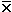Genetic Drift

Genetic Drift is stochasticq [unpredictable, random]
(cf. deterministicq [predictable, due to selection, mutation, and (or) migration]

Sewall Wright (1889 - 1988): "Evolution and the Genetics of Populations" (1931)

Stochasticq greater than deterministicq in small populations:
allele frequencies drift more in 'small' than 'large' populations

Drift most noticeable if s 0, and (or) N small (< 10) and (or)  N 1/s
Wright - Fisher Model for haploid organisms, finite size N (or 2N ~ diploid), discrete generations [NS 02-01smc]

q drifts between generations (variation decreases within populations over time);
eventually, allele is lost (q = 0) or fixed (q = 1)

Ex: Matlab model of Cepaea nemoralis

q drifts among populations (variance increases among populations over time) [NS 02-02smc]
eventually, half lose the allele, half fix it

**=> Variation 'fixed' or 'lost'  &  populations diverge due to chance <=**

Evolutionary significance
"Gambler's Dilemma" : if you play long enough, you win or lose everything
All populations finite: many very small, somewhere or sometime
Evolution occurs on vast time scales: "one in a million chance" a certainty (or a rate)
Reproductive success of individuals in variable: "The race is not to the swift ..."

What happens in the really long run?

Effective Population Size (Ne)
size of 'ideal' population Nexp with same [evolutionary / population genetic] behavior as 'real' population with Nobs
same genetic variation (measured as Hobs)
same inbreeding coefficient F [see derivation]
same 'genetic drift' (measured as genetic variance)
in any single generation
σ2 = (pq)/2N

${\displaystyle V_{t}\approx pq\left(1-\exp \left(-{\frac {t}{2N_{e}}}\right)\right)}$over time t~ approximately the number of breeding individuals in the local population

Consider four special cases where Ne << Nobs  [the observed 'count' of individuals]:

(1) Unequal sex ratio

Ne = (4)(Nm)(Nf) / (Nm + Nf)    [see derivation]

where Nm & Nf = counts of breeding males & females, respectively

"harem" structures in mammals (Nm << Nf)
Ex.: if Nm  = 1 "Alpha Male" and  Nf = 200
then     Ne = (4)(1)(200)/(1 + 200) 4

A single male elephant seal (Mirounga) does most of the breeding [NS 02-04]
[Elephant seals have very low genetic variation]

eusocial (colonial) insects like ant & bees (Nf << Nm)
Ex.: if Nf = 1 "queen" and  Nm= 1,000 drones
then     Ne = (4)(1)(1,000)/(1 + 1,000) 4
Hives are very small families

(2) Unequal reproductive success
In stable population, Noffspring / parent = 1
"Random" reproduction follows Poisson distribution (N = 11):
Characteristics:=
σ2 ; if= 1,  σ2 = σ
~ 1/3 of (female) parents have 0, ~1/3 have 1, ~1/3 have 2 or more

 N σ Ne = Reproductive strategy 1 1 Nobs Random breeding success 1 0 2x Nobs A conservation strategy 1 > 1 < Nobs K-strategy, as in Homo 1 >> 1 << Nobs r-strategy, as in Gadus

Conservation strategy: All animals bred equally, gene pool conserved
K
-strategy
: keep N constant near carrying capacity (K) by investing parental care
r-strategy: maximize chance of success with high reproductive rate (r),
little parental investment

(3) Population size variation over time

Ne = harmonic mean of N  = inverse of arithmetic mean of inverses
[much closer to smallest value in series]
n
Ne = n /  [ Σ(1/Ni) ]  where Ni = pop size in i th generation
i=1

Populations exist in fluctuating environments:
Populations unlikely to be stable over very long periods of time:
101~2 forest fire , 102~3 flood , 10-4 ice age

Ex.: if typical N = 1,000,000  & every 100th generation 'bottlenecks' to N = 10 :
then  Ne = (100) / [(99)(10-6) + (1)(1/10)]100 / 0.1 = 1,000 [HOMEWORK]

Founder Effect & Bottlenecks:
Populations started by (very) small number of individuals,
or undergo dramatic reduction in size [NS 02-03]

Ex.: Founder Origin of Newfoundland moose (Alces):
2 bulls + 2 cows at Howley in 1904
[1 bull + 1 cow at Gander in 1878 didn't succeed].

Ex.: Bottlenecks in population cycles: Hudson Bay Co. trapping records (Elton 1925)
Population densities of lynx, hare, muskrat cycle over several orders of magnitude
Explained as Lynx cycle "chasing" hare cycle

(4) Non-uniform population dispersion

For a population with a landscape architecture defined as

= density = # individuals / unit area

σ = mean dispersion between adult & offspring birthplace
(if normally distributed, then variance =
σ2 )

Ne = (4π)ẟσ2

Ex.: In old-field Deer Mice (Peromyscus maniculatus),

ẟ = 6 / 104 m-2, σ = 114 m, so σ2 = 1.3x104 m2
Ne = 98 / "neighborhood"
[cf: Canadian Rules football field = 6x103 m2:
= 3.4 mice / field

BUT if mice live in barns at 4X the density & 1/10 the dispersion:
Ne = 4

The effect of drift on genetic variation in populations

Larger populations more variable (higher H) than smaller
if s = 0Heterozygosity (H) reflects balance between
loss of alleles by drift and replacement by mutation

The Neutral Equation: H = (4Neµ) / (4Neµ + 1)
Ex.: if µ= 10-6   & Ne = 106   then   Neµ = 1   and Hexp = (4)/(4 + 1) = 0.80
But for protein electrophoretic data, typical Hobs0.20  which suggests  Ne105

Natural populations have much smaller Ne than observed Nc count

Stochastic effects may often be more important than deterministic processes in evolution.

Text material © 2021 by Steven M. Carr